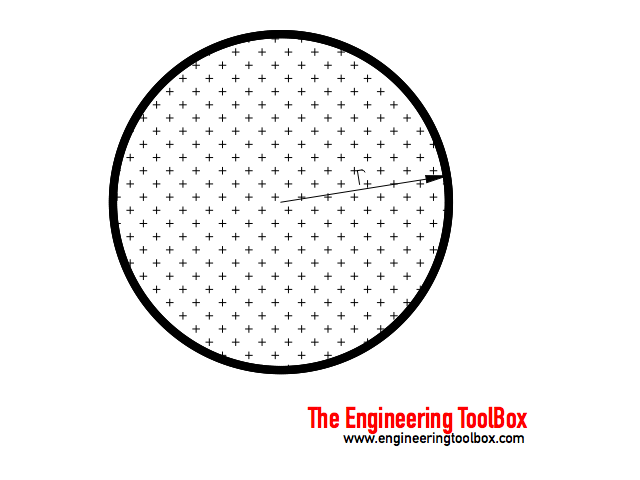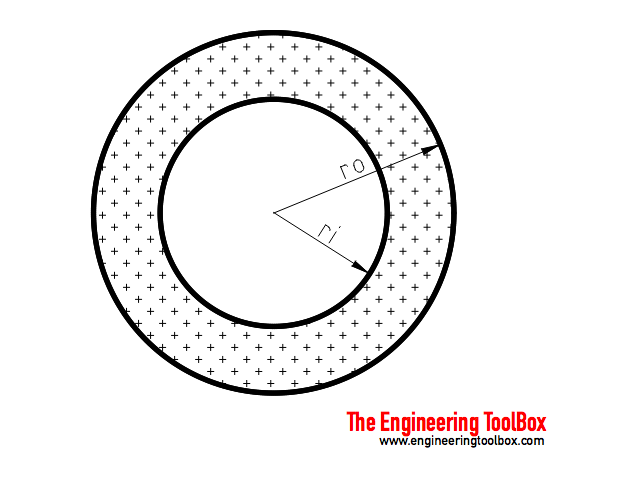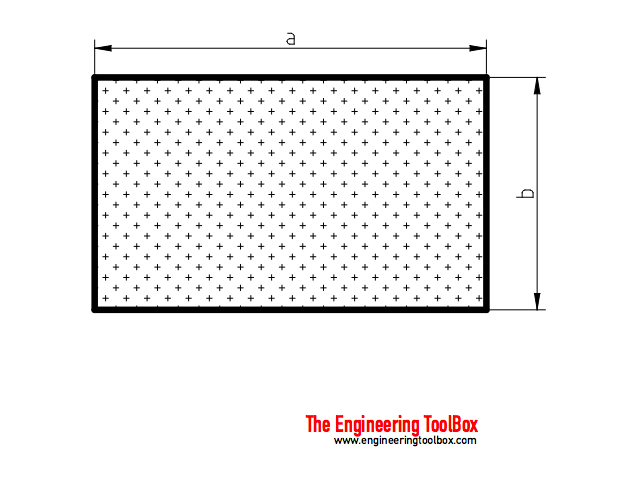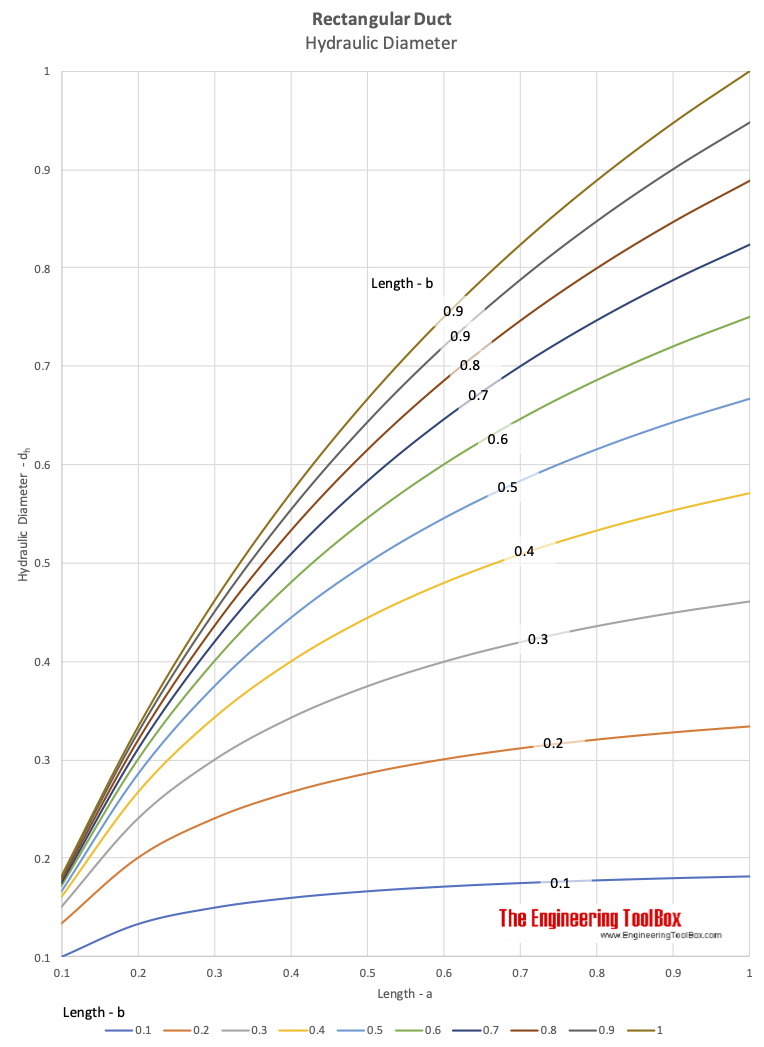Engineering ToolBox - Resources, Tools and Basic Information for Engineering and Design of Technical Applications!

# Fluid Flow - Hydraulic Diameter

## Calculate hydraulic diameter for pipes and ducts.

Hydraulic diameter - dh - is the "characteristic length" used to calculate the dimensionless Reynolds Number to determine if a flow is turbulent or laminar. A flow is

• laminar if Re < 2300
• transient for 2300 < Re < 4000
• turbulent if Re > 4000

Note that the velocity in the Reynolds equation is based on actual cross section area of duct or pipe.

The hydraulic diameter is used the Darcy-Weisbach equation to calculate pressure loss in ducts or pipes.

Note! - the hydraulic diameter is not the same as the geometrical equivalent diameter of non-circular ducts or pipes.

Hydraulic diameter can be calculated with the generic equation

dh = 4 A / p                               (1)

where

dh = hydraulic diameter (m, ft)

A = area section of the duct or pipe (m2, ft2)

p = "wetted" perimeter of the duct or pipe (m, ft)

Note! - inches are commonly used in the Imperial unit system

### Hydraulic Diameter of a Circular Tube or DuctBased on equation (1) the hydraulic diameter of a circular duct can be expressed as:

dh = 4 π r2 / 2 π r

= 2 r

= d                                  (2)

where

r = pipe or duct inside radius  (m, ft)

d = pipe or duct inside diameter  (m, ft)

As we could expect the hydraulic diameter of a standard circular tube or duct is the inner diameter or two times the inner radius.

### Hydraulic Diameter of Circular Tube with Circular Tube on the Inside

The flow is in the volume between the inside and outside pipe.Based on equation (1) the hydraulic diameter of a circular duct or tube with an inside duct or tube can be expressed as

dh = 4 (π ro2 - π ri2) / (2 π ro + 2 π ri)

= 2 (ro - ri)                                        (3)

where

ro = inside radius of the outside tube (m, ft)

ri = outside radius of the inside tube (m, ft)

### Hydraulic Diameter of Rectangular Tubes or DuctsBased on equation (1) the hydraulic diameter of a rectangular duct or pipe can be calculated as

dh = 4 a b / (2 (a + b))

= 2 a b / (a + b)                                 (4)

where

a = width/height of the duct (m, ft)

b = height/width of the duct (m, ft)

### Related Mobile Apps from The EngineeringToolBox

- free apps for offline use on mobile devices.

#### Rectangular to Circulate Duct/Tube Hydraulic Diameter Calculator

The calculator below is based on formula (4) an can be used to calculate the hydraulic diameter of rectangular duct or tube. The formula is generic and any unit can be used.

Length side a - (m, ft)

Length side - b - (m, ft)### Equivalent diameter

Note! The hydraulic diameter is not the same as the equivalent diameter. The equivalent diameter is the diameter of a circular duct or pipe that gives the same pressure loss as a rectangular duct or pipe.

## Related Topics

• ### Fluid Flow and Pressure Loss

Pipe lines - fluid flow and pressure loss - water, sewer, steel pipes, pvc pipes, copper tubes and more.
• ### Fluid Mechanics

The study of fluids - liquids and gases. Involving velocity, pressure, density and temperature as functions of space and time.
• ### Ventilation

Systems for ventilation and air handling - air change rates, ducts and pressure drops, charts and diagrams and more.

## Related Documents

• ### Air Ducts - Friction Loss Diagram

A major friction loss diagram for air ducts - Imperial units ranging 10 - 100 000 cfm.
• ### Air Ducts - Major Friction Head Loss, Online Calculator

Head loss or major loss in air ducts - equations and online calculator for rectangular and circular ducts - Imperial and SI units.
• ### Colebrook Equation

Friction loss coefficients in pipes, tubes and ducts.
• ### Darcy-Weisbach Equation - Major Pressure and Head Loss due to Friction

The Darcy-Weisbach equation can be used to calculate the major pressure and head loss due to friction in ducts, pipes or tubes.
• ### Duct Velocity

Calculate velocities in circular and rectangular ducts - imperial and SI-units - online calculator.
• ### Ducts - Cross-Sectional Area vs. Diameter

Circular ducts sross-sectional areas.
• ### Energy Equation - Pressure Loss vs. Head Loss

Calculate pressure loss - or head loss - in ducts, pipes or tubes.
• ### Equivalent Length vs. Minor Pressure Head Loss in Pipe and Duct Components

Minor pressure and head loss in pipes vs. equivalent length in tubes and duct systems.
• ### Flow Section Channels - Geometric Relationships

Geometric relationships like area, wetted perimeter and hydraulic diameter.
• ### Fluid Flow - Equivalent Diameter

Convert rectangular and oval duct geometry to a equivalent circular diameter - online calculator with imperial and SI-units.
• ### Hazen-Williams Pressure Loss Equation

The Hazen-Williams equation can be used to calculate the pressure drop (psi) or friction loss in pipes or tubes.
• ### Pipe and Duct Systems - Total Head Loss

Major and minor loss in pipes, tubes and duct systems.
• ### Rectangular Ducts - Hydraulic Diameter

Calculate hydraulic diameter for rectangular ducts - metric units.
• ### Reynolds Number

Introduction and definition of the dimensionless Reynolds Number - online calculators.
• ### Water Flow in Tubes - Reynolds Number

Reynolds number for clean cold water flow.

## Engineering ToolBox - SketchUp Extension - Online 3D modeling!

Add standard and customized parametric components - like flange beams, lumbers, piping, stairs and more - to your Sketchup model with the Engineering ToolBox - SketchUp Extension - enabled for use with older versions of the amazing SketchUp Make and the newer "up to date" SketchUp Pro . Add the Engineering ToolBox extension to your SketchUp Make/Pro from the Extension Warehouse !

We don't collect information from our users. More about

## Citation

• The Engineering ToolBox (2003). Fluid Flow - Hydraulic Diameter. [online] Available at: https://www.engineeringtoolbox.com/hydraulic-equivalent-diameter-d_458.html [Accessed Day Month Year].

Modify the access date according your visit.

9.19.12